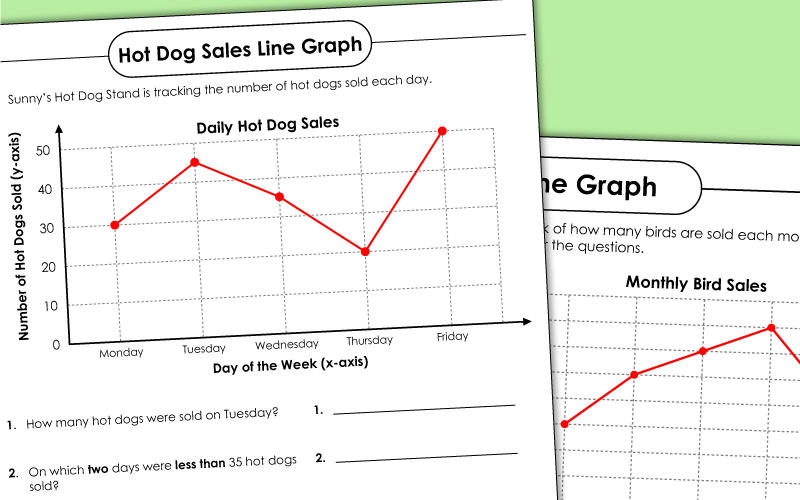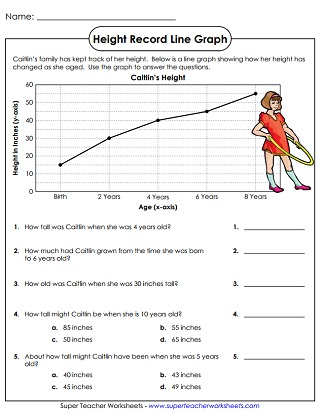# Line Graph Worksheets

A line graph is used to show data over a period of time.  We have a collection of printable line graph worksheets for you to use with your students.## Basic Line Graphs

Graph of a child's height as they grow from birth to age 8; Scales count by 10sand 2s; Grades 2-3
Students make a line graph showing the number birds sold at Steve's Pet World; Grades 2-3
Line graph shows the number of stamps in a collection; Grades 2-3

## Intermediate Line Graphs

Air temperature line graph over a 1-month period; scales count by 3s and 7s; Grades 4 and up
A line graph of air temperature as it changes over a 1-day period. This worksheet uses the Fahrenheit scale.
A line graph shows how air temperature changes during a 1-day period. This graph uses the Celsius temperature scale.

Line graph shows the number of rolls sold at Pan's bakery; Students must convert to and from dozens; Grades 4 and up

Pictograph Worksheets

Bar Graph Worksheets

Printable bar graph worksheets with questions

Pie Graph Worksheets

A collection of circle graph (pie graph) worksheets

Line Plot Worksheets

Print these line plot worksheets to teach frequency distribution of numbers.

## Worksheet ImagesMy Account
Site Information